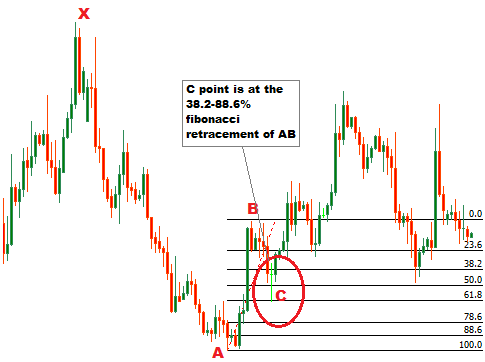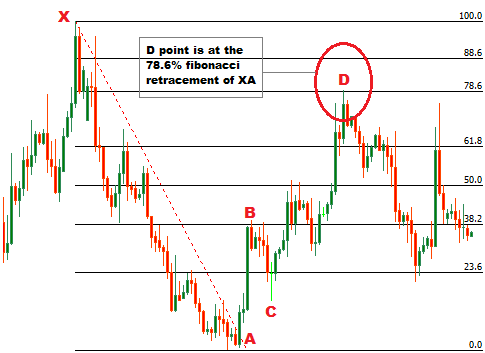Welcome! Dear Traders,you are reading my forex trading experiences. Forex trading is a very profitable and very risky business opportunity. If you are a beginner, calm down,have a cup of coffee, and convince yourself that you need to study hard to win in forex trading. Obviously, the task is not easy as the statistics claim that only 5% traders win in forex trading. If you are determined, serious,and hard working, you can surely be included in the group of winners.

## Wednesday, August 5, 2015

### HOW TO TRADE BEARISH BAT HARMONIC CHART PATTERN

Bearish bat harmonic chart pattern suggests a short entry upon completion of the pattern. This post explains the way to find or draw a bearish bat harmonic chart pattern, and the way to trade a bearish bat harmonic chart pattern.In the first part of the post, we will learn to draw the pattern with the fibonacci retracement tool and with out the fibonacci retracement tool.

How to draw a bearish bat harmonic chart pattern on price chart ?
To draw a harmonic chart pattern, first of all we will find the X and A points of the pattern. X and A points of the pattern is found at the top and bottom of a bearish trend respectively. In the second step, we will draw a fibonacci retracement tool from X to A to find the B point of the pattern. The B point should be between the 38.2-50% fibonacci retracement of XA.

To find the B point without fibonacci retracement tool, we will use the following formulas-
(IA) AB= (price at X point-price at A point) X 0.382
(IB) Price level at 38.2% of XA= (result found from formula (IA) + price at point A)
(IIA) AB= (price at X point-price at A point) X 0.50
(IIB) Price level at 50% of XA= (result found from formula (IIA) + price at point A)

The B point of this pattern should lie between the price levels found from formula (IB) and (IIB). This satisfies the AB/XA=0.382-0.50 condition of the pattern. Look at the following illustration.

In the next step, we are going to find the C point of the bearish bat harmonic chart pattern. To find the C point, we will draw a fibonacci retracement tool from A to B. The C point should lie between the 38.2-88.6% fibonacci retracement of AB.

To find the C point without fibonacci retracement tool, we will use the formulas-
(IA) BC= (price at B point-price at A point) X 0.382
(IB) Price level at 38.2% of AB= (price at point B-result found from formula (IA))
(IIA) BC= (price at B point-price at A point) X 0.886
(IIB) Price level at 88.6% of AB= (price at point B-result found from formula (IIA))

The C point of this pattern should lie between the price levels found from formula (IB) and (IIB). This satisfies the BC/AB=0.382-0.886 condition of the pattern. Look at the following illustration.In this step, we will find the D point of the pattern. To find the D point, we will draw a fibonacci retracement tool from point B to point C. The D point should  lie between the 161.8-261.8% fibonacci retracement of BC.

To find the D point without fibonacci retracement tool, we will use the formulas-
(IA) CD= (price at B point-price at C point) X 1.618
(IB) Price level at 161.8% of AB= (price at point C+result found from formula (IA))
(IIA) CD= (price at B point-price at C point) X 2.618
(IIB) Price level at 261.8% of AB= (price at point C+result found from formula (IIA))

The D point of this pattern should lie between the price levels found from formula (IB) and (IIB). This satisfies the CD/BC=1.618-2.618 condition of the pattern. Look at the following illustration.

In case of this harmonic pattern, D point should be reconfirmed to satisfy the condition left. To reconfirm the D point, draw a fibonacci retracement tool from X to A. The D point should lie at the 88.6% fibonacci retracement of XA.

To find the D point without fibonacci retracement tool, we will use the formulas-
(IA) AD= (price at X point-price at A point) X 0.886
(IB) Price level at 88.6% of XA= (price at point A+result found from formula (IA))

The D point of this pattern should lie at the price levels found from formula (IB). This satisfies the AD/XA=0.886 condition of the pattern. Look at the following illustration. You can see, the condition is not fulfilled here, this is because the D point is confirmed at 78.6% fibonacci retracement of XA and 2.618% fibonacci retracement of BC. Two resistance level are lied together at point D here, and 2.618% is the highest limit for the previous step.

Remember, the D point is the entry point of the pattern and it is confirmed by two conditions. A reliable D point should satisfy the both conditions. In this case it fails two meet the second condition  AD/XA=0.886 because it reached the highest limit of the CD/BC=1.618-2.618 condition.How to trade the bearish bat harmonic chart pattern?
A short entry is suggested when the D point of the pattern is confirmed. The stop loss for the order can be placed at X point. The profit target for the order should be placed at the 38.2, 50% or 61.8% fibonacci retracement level of CD or AD.

B and C points can also be traded, if the entries are confirmed by other technical analysis tool(s)

Hope this post will enrich your technical analysis knowledge. If you have any question, you can drop a comment below, and you can subscribe this blog to receive the updates.

Thank You & Good Luck

#### 1 comment:

1.To trade under a bearish forex market it needs some accurate and sure shot forex signals.

Drop a comment...

### HOW CAN WE INTERPRET BIG GREEN CANDLE & BIG RED CANDLE ON CANDLESTICKS CHART

your F X guide- Candlesticks chart patterns are very simple to identify and very effective to trade. Generally, we find two kinds of can...